Question

A radioactive nucleus has a half-life of 5 x 108 years. Assuming that a sample of rock (say, in an asteroid) solidified right after the solar system formed, approximately what fraction of the radioactive element should be left in the rock today?

1.So the present mass will be 0.0017002 times of the initial mass of the sample.

Explanation:

Given:

• half life of the given sample,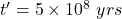• We know that solar system came in to existence approximately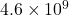years ago.

Let the initial quantity on the formation of the solar system be,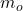Then the final quantity at present be: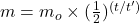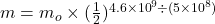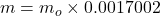So the present mass will be 0.0017002 times of the initial mass of the sample.